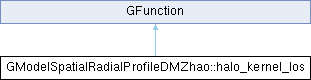GammaLib  1.7.0.dev

#include <GModelSpatialRadialProfileDMZhao.hpp>## Public Member Functions

halo_kernel_los (const double &scale_radius, const double &halo_distance, const double &alpha, const double &beta, const double &gamma, const double &theta, const double &core_radius)

double eval (const double &los)
Kernel for zhao halo density profile squared. More...Public Member Functions inherited from GFunction
GFunction (void)
Void constructor. More...

GFunction (const GFunction &function)
Copy constructor. More...

virtual ~GFunction (void)
Destructor. More...

GFunctionoperator= (const GFunction &function)
Assignment operator. More...

## Protected Attributes

double m_halo_distance

double m_alpha

double m_beta

double m_gamma

double m_thetaProtected Member Functions inherited from GFunction
void init_members (void)
Initialise class members. More...

void copy_members (const GFunction &function)
Copy class members. More...

void free_members (void)
Delete class members. More...

## Detailed Description

Definition at line 97 of file GModelSpatialRadialProfileDMZhao.hpp.

## Constructor & Destructor Documentation

 GModelSpatialRadialProfileDMZhao::halo_kernel_los::halo_kernel_los ( const double & scale_radius, const double & halo_distance, const double & alpha, const double & beta, const double & gamma, const double & theta, const double & core_radius )
inline

Definition at line 99 of file GModelSpatialRadialProfileDMZhao.hpp.

## Member Function Documentation

 double GModelSpatialRadialProfileDMZhao::halo_kernel_los::eval ( const double & los )
virtual

Kernel for zhao halo density profile squared.

Parameters
 [in] los Distance from observer to point in space (meters)
Returns
Halo density.

Computes the value of a zhao halo density profile squared, at distance l from observer, at angle

$\theta$

from the halo center, with a halo posessing a scale radius of

$r_s$

:

$f(\theta, l) = \frac{1}{ g^{\gamma} {\left( g^{\alpha} + 1 \right)}^{ \frac{\beta-\gamma}{\alpha} } }$

where

$g = \frac{ \sqrt{l^2+d^2-2ldCos(\theta)} }{r_s}$

$\beta$

is the slope of the density at radii much bigger than

$r_s$

$\gamma$

is the slope of the density profile at radii much smaller than

$r_s$

$\alpha$

governs how large the transition region is between these two slopes. larger

$\alpha$

means a smaller transition region.

This profile is detailed in: Zhao, 1996 "Analytical models for galactic nuclei" Monthly Notices of the Royal Astronomical Society, 278, 488-49 http://mnras.oxfordjournals.org/content/278/2/488.short

Implements GFunction.

Definition at line 696 of file GModelSpatialRadialProfileDMZhao.cpp.

References cos(), m_alpha, m_beta, m_core_radius, m_gamma, m_halo_distance, m_scale_radius, m_theta, pow(), and sqrt().

## Member Data Documentation

protected

Definition at line 117 of file GModelSpatialRadialProfileDMZhao.hpp.

Referenced by eval().

protected

Definition at line 118 of file GModelSpatialRadialProfileDMZhao.hpp.

Referenced by eval().

protected

Definition at line 121 of file GModelSpatialRadialProfileDMZhao.hpp.

Referenced by eval().

protected

Definition at line 119 of file GModelSpatialRadialProfileDMZhao.hpp.

Referenced by eval().

protected

Definition at line 116 of file GModelSpatialRadialProfileDMZhao.hpp.

Referenced by eval().

protected

Definition at line 115 of file GModelSpatialRadialProfileDMZhao.hpp.

Referenced by eval().